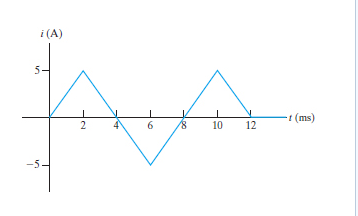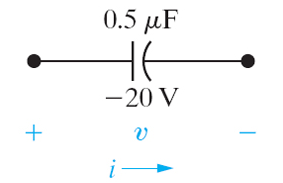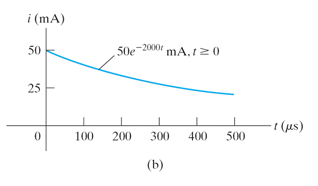Create an Account

Home / Questions / 1The current in a 150 H inductor is known to be iL25te500tAfort 0 where t is in seconds A...

1The current in a 150 H inductor is known to be iL25te500tAfort 0 where t is in seconds Assume the passive sign convention 1 1Find the voltage across the inductor for t 0 Express your

1.The current in a 150 ?H inductor is known to be iL=25te^?500tAfort?0, where t is in seconds. Assume the passive sign convention.

1.1Find the voltage across the inductor for t>0.

1.2

Find the power at the terminals of the inductor when t = 6.7ms .

2.2.1Find the voltage on the capacitor at t = 10 ms.

2.2How much energy is stored in the capacitor by this current?

3.The initial voltage on the 0.5 ?F capacitor shown in the figure (Figure 1) is ?20V. The capacitor current has the waveform shown in the figure (Figure 2) .

How much energy is stored in the capacitor at t = 585?s ?

Show transcribed image text 1.The current in a 150 ?H inductor is known to be iL=25te^?500tAfort?0, where t is in seconds. Assume the passive sign convention. 1.1Find the voltage across the inductor for t>0. Express your answer in terms of t, where t is in milliseconds. Express your answer using three significant figures. 1.2 Find the power at the terminals of the inductor when t = 6.7ms . 2. The initial voltage on the 0.5 ?F capacitor shown in the figure (Figure 1) is ?20V. The capacitor current has the waveform shown in the figure (Figure 2) . How much energy is stored in the capacitor at t = 585?s ? 2.1Find the voltage on the capacitor at t = 10 ms. 2.2How much energy is stored in the capacitor by this current? 3.

Jul 27 2020 View more View LessSubscribe To Get Solution